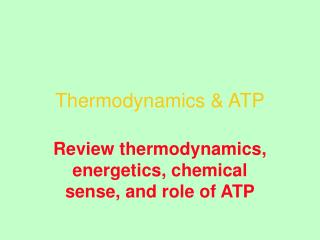DownloadDownload PresentationThermodynamics & ATP

# Thermodynamics & ATP

Télécharger la présentation## Thermodynamics & ATP

- - - - - - - - - - - - - - - - - - - - - - - - - - - E N D - - - - - - - - - - - - - - - - - - - - - - - - - - -
##### Presentation Transcript

1. Thermodynamics & ATP Review thermodynamics, energetics, chemical sense, and role of ATP

2. Lecture 24 Thermodynamics in Biology

3. A Simple Thought Experiment

4. Driving Forces for Natural Processes • Enthalpy • Tendency toward lowest energy state • Form stablest bonds • Entropy • Tendency to maximize randomness

5. Enthalpy and Bond Strength • Enthalpy = ∆H = heat change at constant pressure • Units • cal/mole or joule/mole • 1 cal = 4.18 joule • Sign • ∆H is negative for a reaction that liberates heat

6. Entropy and Randomness

7. Entropy and Randomness • Entropy = S = measure of randomness • cal/deg·mole • T∆S = change of randomness • For increased randomness, sign is “+”

8. “System” Definition

9. “System” Definition

10. “System” Definition

11. Cells and Organisms: Open Systems • Material exchange with surroundings • Fuels and nutrients in (glucose) • By-products out (CO2) • Energy exchange • Heat release (fermentation) • Light release (fireflies) • Light absorption (plants)

12. 1st Law of Thermodynamics • Energy is conserved, but transduction is allowed • Transduction

13. 2nd Law of Thermodynamics • In all spontaneous processes, total entropy of the universe increases

14. 2nd Law of Thermodynamics • ∆Ssystem + ∆Ssurroundings = ∆Suniverse > 0 • A cell (system) can decrease in entropy only if a greater increase in entropy occurs in surroundings • C6H12O6 + 6O2  6CO2 + 6H2O complex simple

15. Entropy: A More Rigorous Definition • From statistical mechanics: • S = k lnW • k = Boltzmann constant = 1.3810–23 J/K • W = number of ways to arrange the system • S = 0 at absolute zero (-273ºC)

16. Gibbs Free Energy • Unifies 1st and 2nd laws • ∆G • Gibbs free energy • Useful work available in a process • ∆G = ∆H – T∆S • ∆H from 1st law • Kind and number of bonds • T∆S from 2nd law • Order of the system

17. ∆G • Driving force on a reaction • Work available  distance from equilibrium • ∆G = ∆H – T∆S • State functions • Particular reaction • T • P • Concentration (activity) of reactants and products

18. Equilibrium • ∆G = ∆H – T∆S = 0 • So ∆H = T∆S • ∆H is measurement of enthalpy • T∆S is measurement of entropy • Enthalpy and entropy are exactly balanced at equilibrium

19. Effects of ∆H and ∆S on ∆G Voet, Voet, and Pratt. Fundamentals of Biochemistry. 1999.

20. Standard State and ∆Gº • Arbitrary definition, like sea level • [Reactants] and [Products] • 1 M or 1 atmos (activity) • T = 25ºC = 298K • P = 1 atmosphere • Standard free energy change = ∆Gº

21. Biochemical Conventions: ∆Gº • Most reactions at pH 7 in H2O • Simplify ∆Gº and Keq by defining [H+] = 10–7 M • [H2O] = unity • Biochemists use ∆Gº and Keq

22. Relationship of ∆G to ∆Gº • ∆G is real and ∆Gº is standard • For A in solution • GA = GA + RT ln[A] • For reaction aA + bB  cC + dD • ∆G = ∆Gº + RT ln • Constant Variable (from table) º [C]c [D]d } [A]a [B]b

23. Relationship Between ∆Gº and Keq [C]c [D]d • ∆G = ∆Gº + RT ln • At equilibrium, ∆G = 0, so • ∆Gº = –RT ln • ∆Gº = –RT ln Keq [A]a [B]b [C]c [D]d [A]a [B]b

24. Relationship Between Keq and ∆Gº

25. Will Reaction Occur Spontaneously? • When: • ∆G is negative, forward reaction tends to occur • ∆G is positive, back reaction tends to occur • ∆G is zero, system is at equilibrium ∆G = ∆Gº + RT ln [C]c [D]d [A]a [B]b

26. A Caution About ∆Gº • Even when a reaction has a large, negative ∆Gº, it may not occur at a measurable rate • Thermodynamics • Where is the equilibrium point? • Kinetics • How fast is equilibrium approached? • Enzymes change rate of reactions, but do not change Keq

27. Reaction A  B B  C Sum: A  C Also: B  A Free energy change ∆G1º ∆G2º ∆G1º + ∆G2º – ∆G1º ∆Gº is Additive (State Function)

28. Glucose + HPO42–  Glucose-6-P ATP  ADP + HPO42– ATP + Glucose  ADP + Glucose-6-P ∆Gº kcal/mol kJ/mol +3.3 +13.8 –7.3 –30.5 –4.0 –16.7 Coupling Reactions

29. Resonance Forms of Pi – – – –

30. Phosphate Esters and Anhydrides

31. Hydrolysis of Glucose-6-Phosphate ∆Gº = –3.3 kcal/mol = –13.8 kJ/mol

32. High ∆Gº Hydrolysis Compounds ∆Gº = –14.8 kcal/mol = –61.9 kJ/mol

33. High ∆Gº Hydrolysis Compounds ∆Gº = –11.8 kcal/mol = –49.3 kJ/mol

34. High ∆Gº Hydrolysis Compounds ∆Gº = –10.3 kcal/mol = –43 kJ/mol

35. Phosphate Anhydrides (Pyrophosphates) ∆Gº = –7.3 kcal/mol = –30.5 kJ/mol

36. Thiol Esters ∆Gº = –7.5 kcal/mol = –31.4 kJ/mol

37. Thiol Esters Thiol ester less resonance-stabilized

38. “High-Energy” Compounds • Large ∆Gº hydrolysis • Bond strain (electrostatic repulsion) in reactant • ATP • Products stabilized by ionization • Acyl-P • Products stabilized by isomerization • PEP • Products stabilized by resonance • Creatine-P

39. “High-Energy” Compounds • “High-energy” compound is one with a ∆Gº below –6 kcal/mol (–25 kJ/mol)

40. High-Energy Compounds

41. Group Transfer Potential